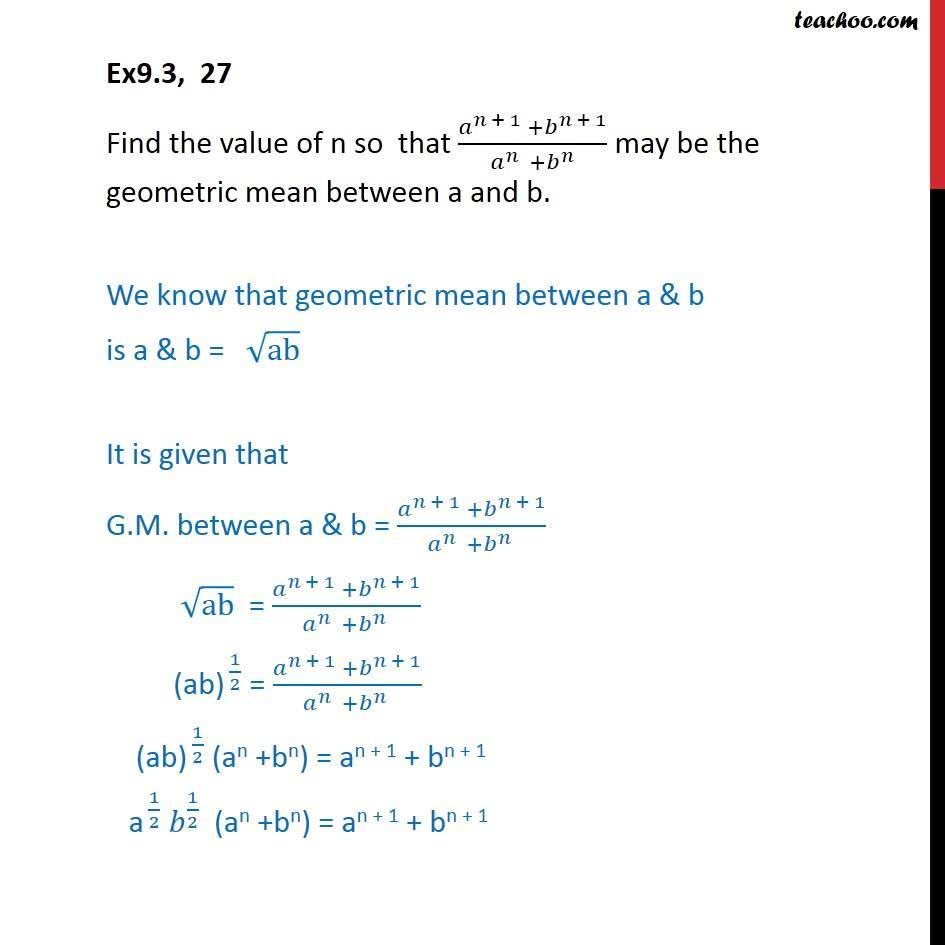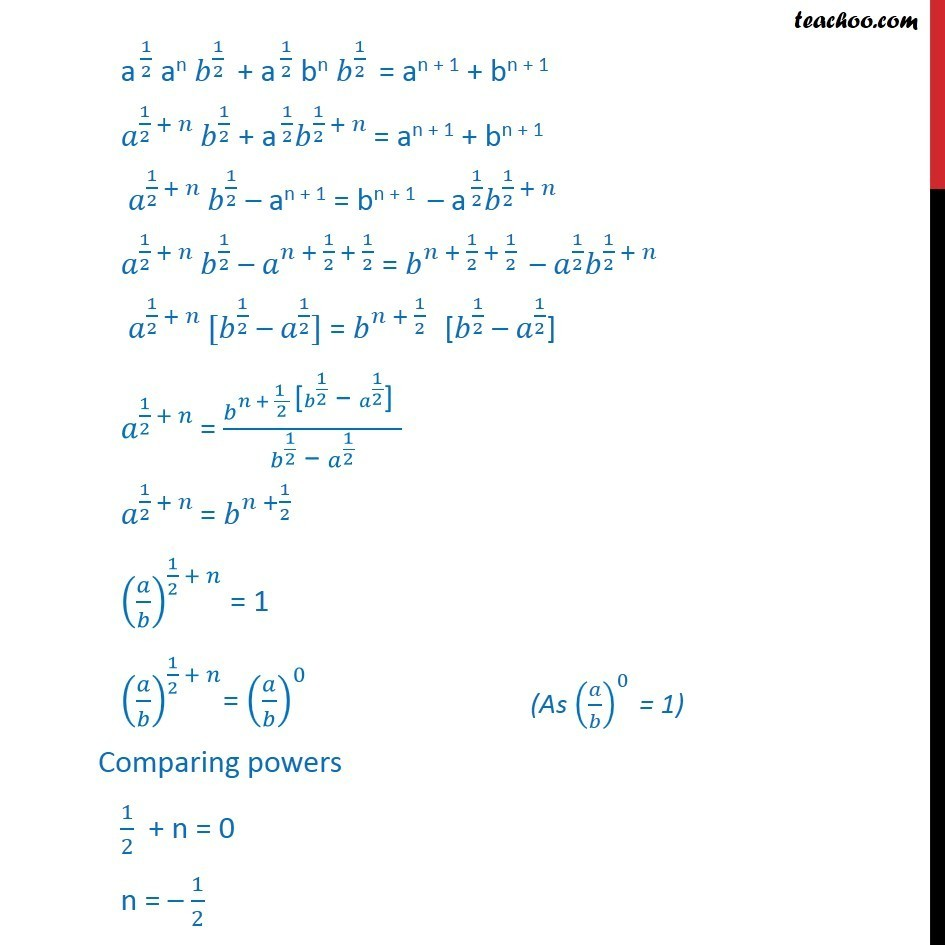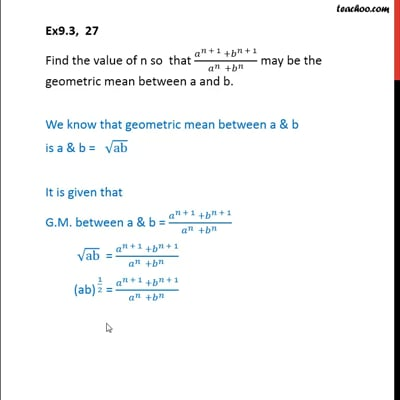Geometric Mean (GM)

Chapter 9 Class 11 Sequences and Series
Concept wiseThis video is only available for Teachoo black users

Introducing your new favourite teacher - Teachoo Black, at only ₹83 per month

### Transcript

Ex9.3, 27 Find the value of n so that (𝑎^(𝑛 + 1) +𝑏^(𝑛 + 1))/(𝑎^(𝑛 ) +𝑏^𝑛 ) may be the geometric mean between a and b. We know that geometric mean between a & b is a & b = √ab It is given that G.M. between a & b = (𝑎^(𝑛 + 1) +𝑏^(𝑛 + 1))/(𝑎^(𝑛 ) +𝑏^(𝑛 ) ) √ab = (𝑎^(𝑛 + 1) +𝑏^(𝑛 + 1))/(𝑎^(𝑛 ) +𝑏^(𝑛 ) ) 〖"(ab)" 〗^(1/2) = (𝑎^(𝑛 + 1) +𝑏^(𝑛 + 1))/(𝑎^(𝑛 ) +𝑏^(𝑛 ) ) 〖"(ab)" 〗^(1/2) (an +bn) = an + 1 + bn + 1 〖"a" 〗^(1/2) 𝑏^(1/2) (an +bn) = an + 1 + bn + 1 〖"a" 〗^(1/2) an 𝑏^(1/2) + 〖"a" 〗^(1/2) bn 𝑏^(1/2) = an + 1 + bn + 1 𝑎^(1/2 + 𝑛 ) 𝑏^(1/2) + 〖"a" 〗^(1/2) 𝑏^(1/2 + 𝑛 )= an + 1 + bn + 1 𝑎^(1/2 + 𝑛 ) 𝑏^(1/2) – an + 1 = bn + 1 – 〖"a" 〗^(1/2) 𝑏^(1/2 + 𝑛 ) 𝑎^(1/2 + 𝑛 ) 𝑏^(1/2) – 𝑎^(𝑛 + 1/2 + 1/2) = 𝑏^(𝑛 + 1/2 + 1/2) – 𝑎^(1/2) 𝑏^(1/2 + 𝑛 ) 𝑎^(1/2 + 𝑛 ) [𝑏^(1/2) – 𝑎^(1/2)] = 𝑏^(𝑛 + 1/2 ) [𝑏^(1/2) – 𝑎^(1/2)] 𝑎^(1/2 + 𝑛 )= 𝑏^(𝑛 + (1 )/2 "[" 𝑏^(1/2) " − " 𝑎^(1/2) "] " )/(𝑏^(1/2) " − " 𝑎^(1/2) ) 𝑎^(1/2 + 𝑛 )= 𝑏^(𝑛 +1/2) (𝑎/𝑏)^(1/2 + 𝑛) = 1 (𝑎/𝑏)^(1/2 + 𝑛)= (𝑎/𝑏)^0 Comparing powers 1/2 + n = 0 n = – 1/2 Hence value of n is - 1/2